# 基于Python的大数据分析实战篇（一）

## ☀前言：

Hello，大家好！我又回来了，下面我将给大家讲解一下，关于我们Python大数据分析的实战测试，希望大家可以在发现错误后，提出意见，我将立刻做出修改。🙇‍

Python数据分析的流程概括起来主要是：读写、处理计算、分析建模和可视化四个部分。在不同的步骤中会用到不同的Python工具。每一步的主题也包含众多内容。

2、利用Python读写数据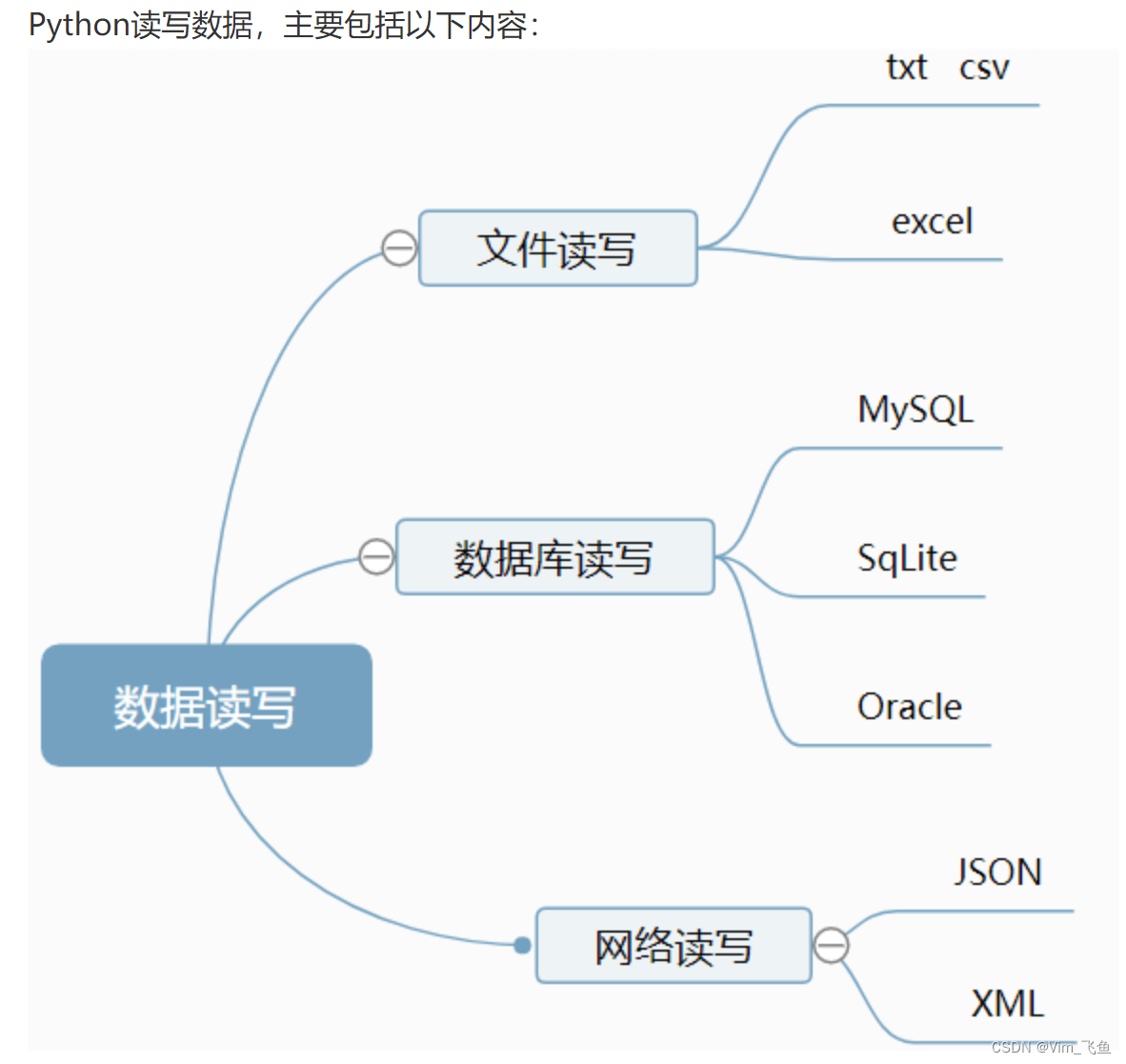3、利用Python处理和计算数据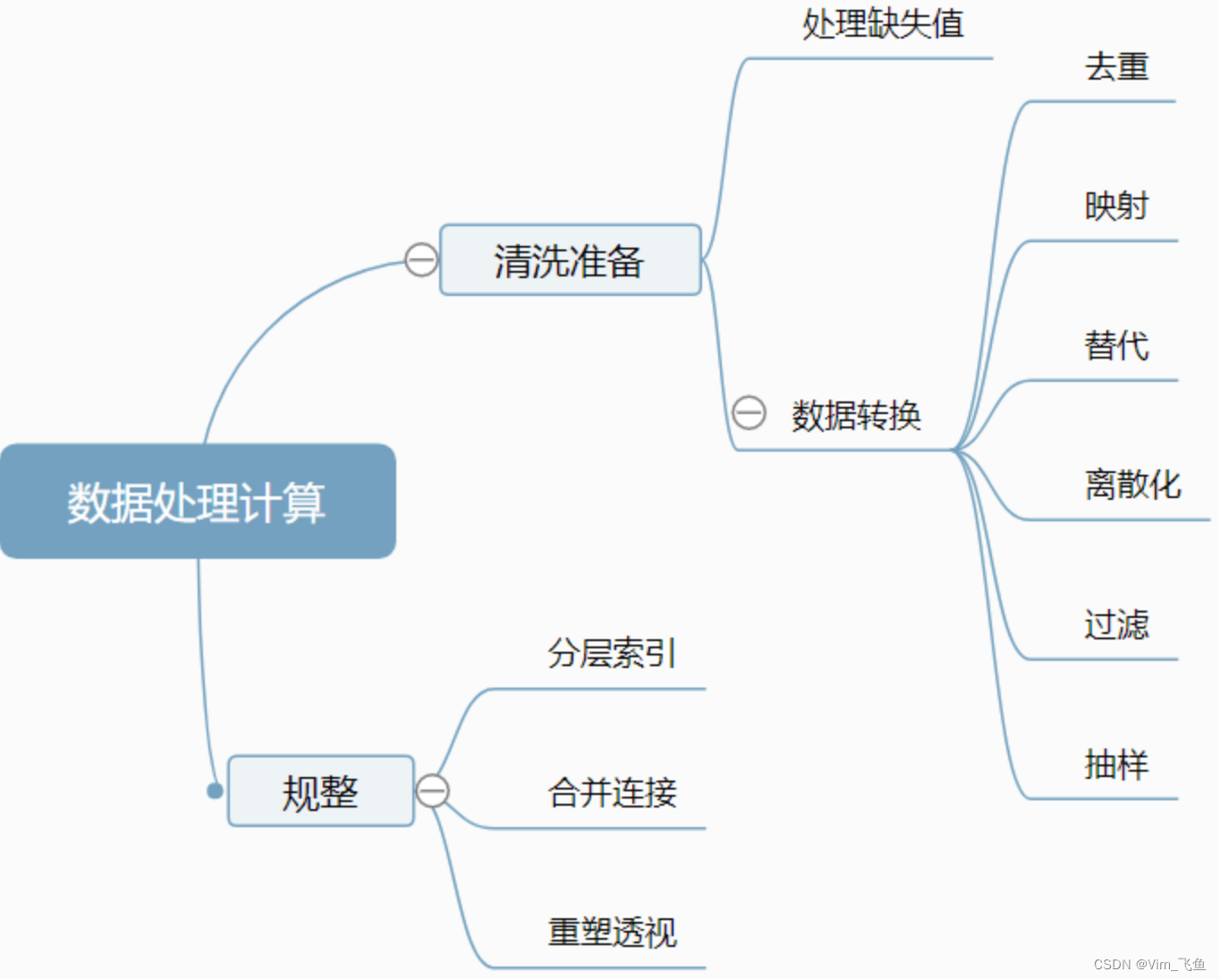4、利用Python分析建模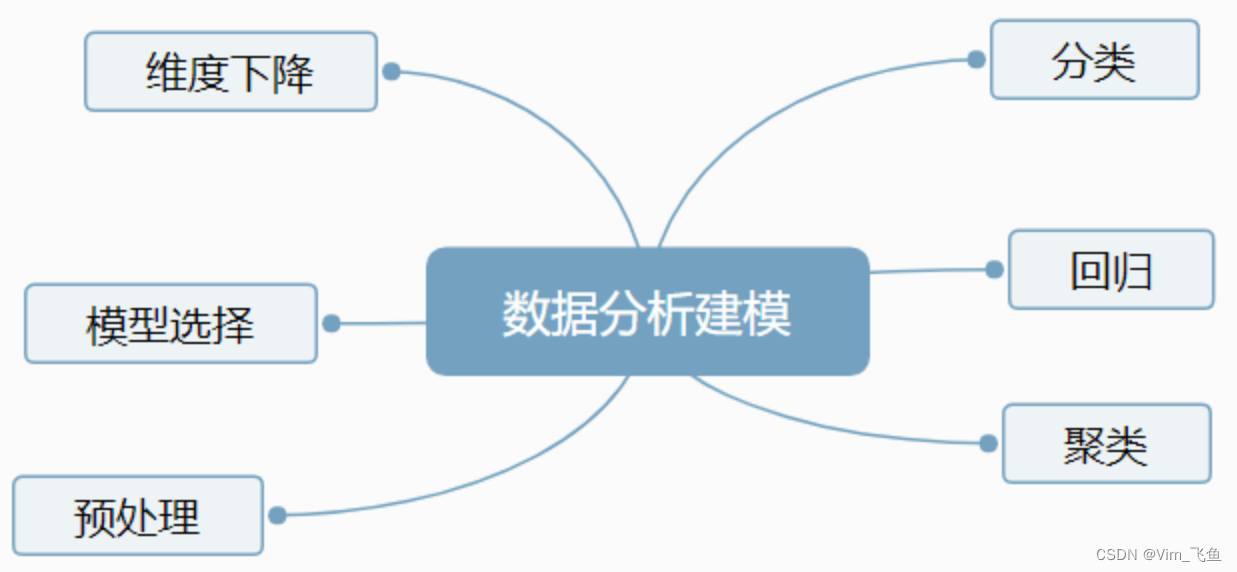5、利用Python数据可视化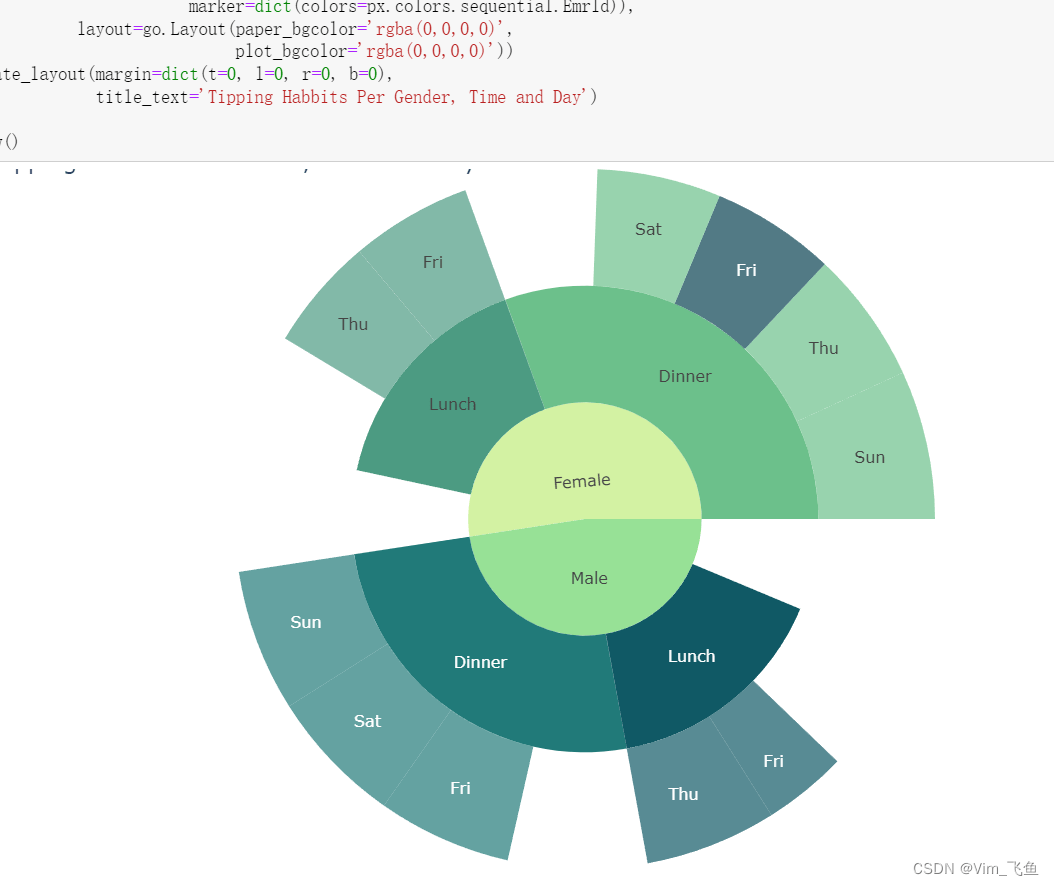以上就是关于Python数据分析的简单介绍，下面步入正题！

### 🐒#1、利用所学知识将文件中的学生成绩信息导入到二维结构中。

``````import numpy as np
import pandas as pd
df``````### 🐒#2、使用numpy求数学的平均分。

``````import numpy as np
import pandas as pd
df
df.iloc[:,7:8]
math=df.iloc[0:99,7:8]
print(math)
print("数学的平均分: ")
math.mean()``````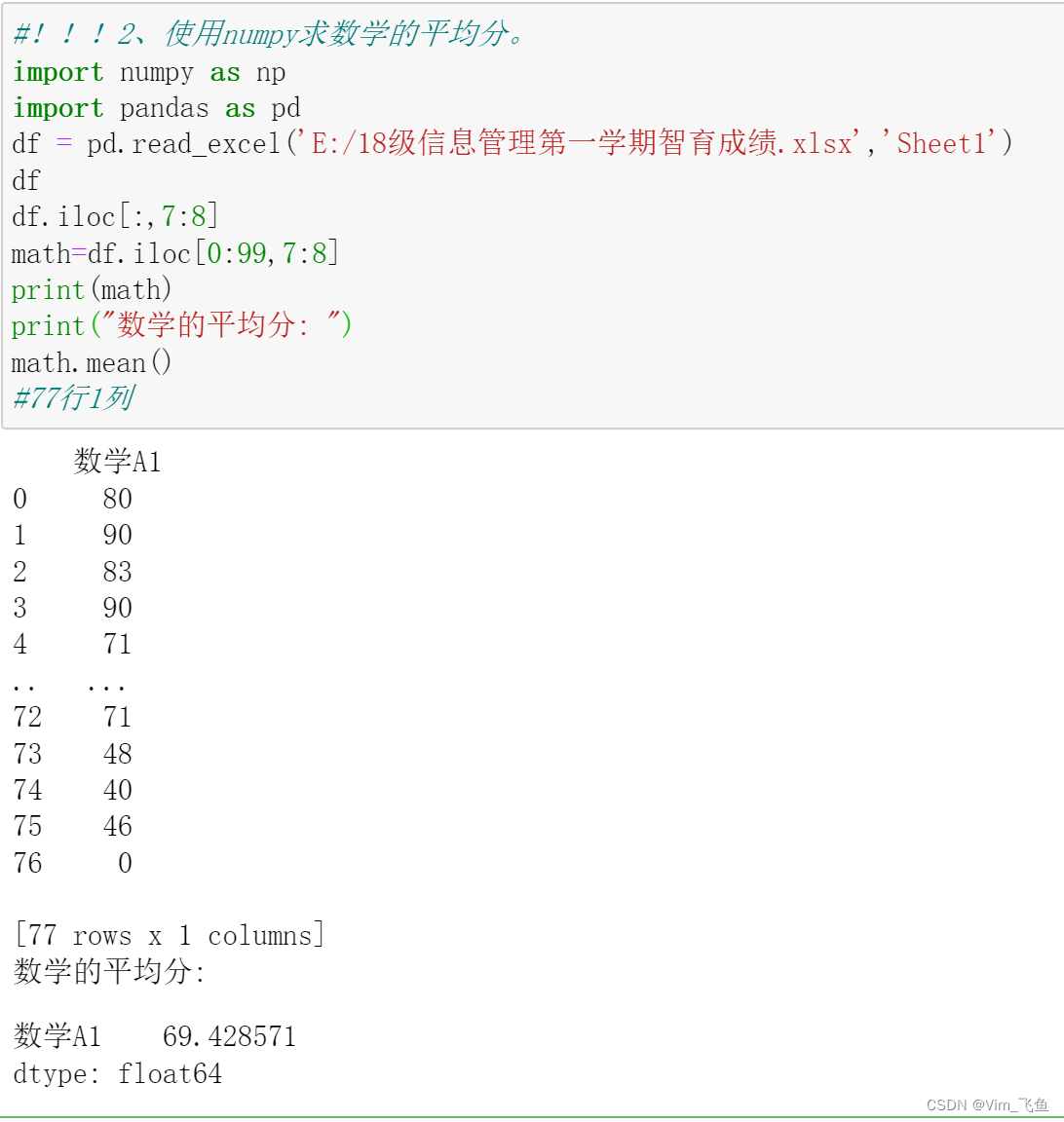### 🐒#3、使用numpy求大学英语的中位数成绩。

``````import numpy as np
import pandas as pd
df
df.iloc[:,5:6]
english=df.iloc[:,5:6]
print(english)
print("英语的中位数: ")
print(np.median(english))``````### 🐒#4、使用numpy找出所有有不及格科目的学生。

``````import numpy as np
import pandas as pd